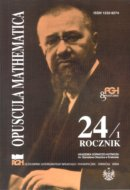Opuscula Math. 24, no. 1 (2004), 43-55

Opuscula Mathematica

# Pm-saturated graphs with minimum size

Aneta Dudek
A. Paweł Wojda

Abstract. By $$P_m$$ we denote a path of order $$m$$. A graph $$G$$ is said to be $$P_m$$-saturated if $$G$$ has no subgraph isomorphic to $$P_m$$ and adding any new edge to $$G$$ creates a $$P_m$$ in $$G$$. In 1986 L. Kászonyi and Zs. Tuza considered the following problem: for given $$m$$ and $$n$$ find the minimum size $$sat(n;P_m)$$ of $$P_m$$-saturated graph and characterize the graphs of $$Sat(n;P_m)$$ - the set of $$P_m$$-saturated graphs of minimum size. They have solved this problem for $$n\geq a_m$$ where $$a_m=\begin{cases}3\cdot 2^{k-1}-2 &\quad\text{ if }\quad m=2k,\, k\gt 2\\ 2^{k+1}-2 &\quad\text{ if }\quad m=2k+1,\, k\geq 2\end{cases}$$. We define $$b_m=\begin{cases}3\cdot 2^{k-2} &\quad\text{ if }\quad m=2k,\, k\geq 3\\ 3\cdot 2^{k-1}-1 &\quad\text{ if }\quad m=2k+1,\, k\geq 3\end{cases}$$ and give $$sat(n;P_m)$$ and $$Sat(n;P_m)$$ for $$m\geq 6$$ and $$b_m\leq n\leq a_m$$.

Keywords: graph, saturated graph, extremal graph.

Mathematics Subject Classification: 05C35.

Full text (pdf)

• Aneta Dudek
• AGH University of Science and Technology, Faculty of Applied Mathematics, al. Mickiewicza 30, 30-059 Cracow, Poland
• A. Paweł Wojda
• AGH University of Science and Technology, Faculty of Applied Mathematics, al. Mickiewicza 30, 30-059 Cracow, Poland